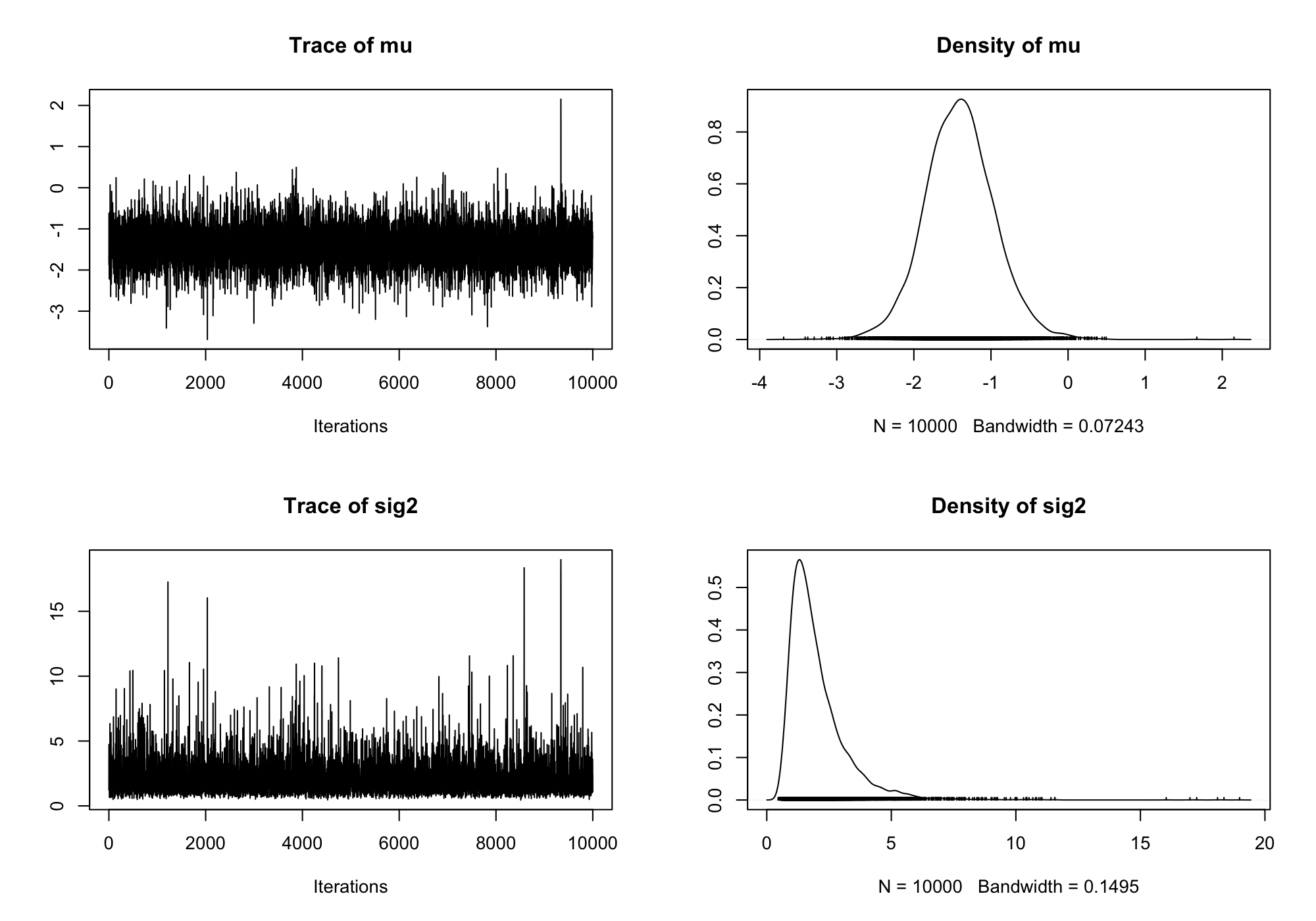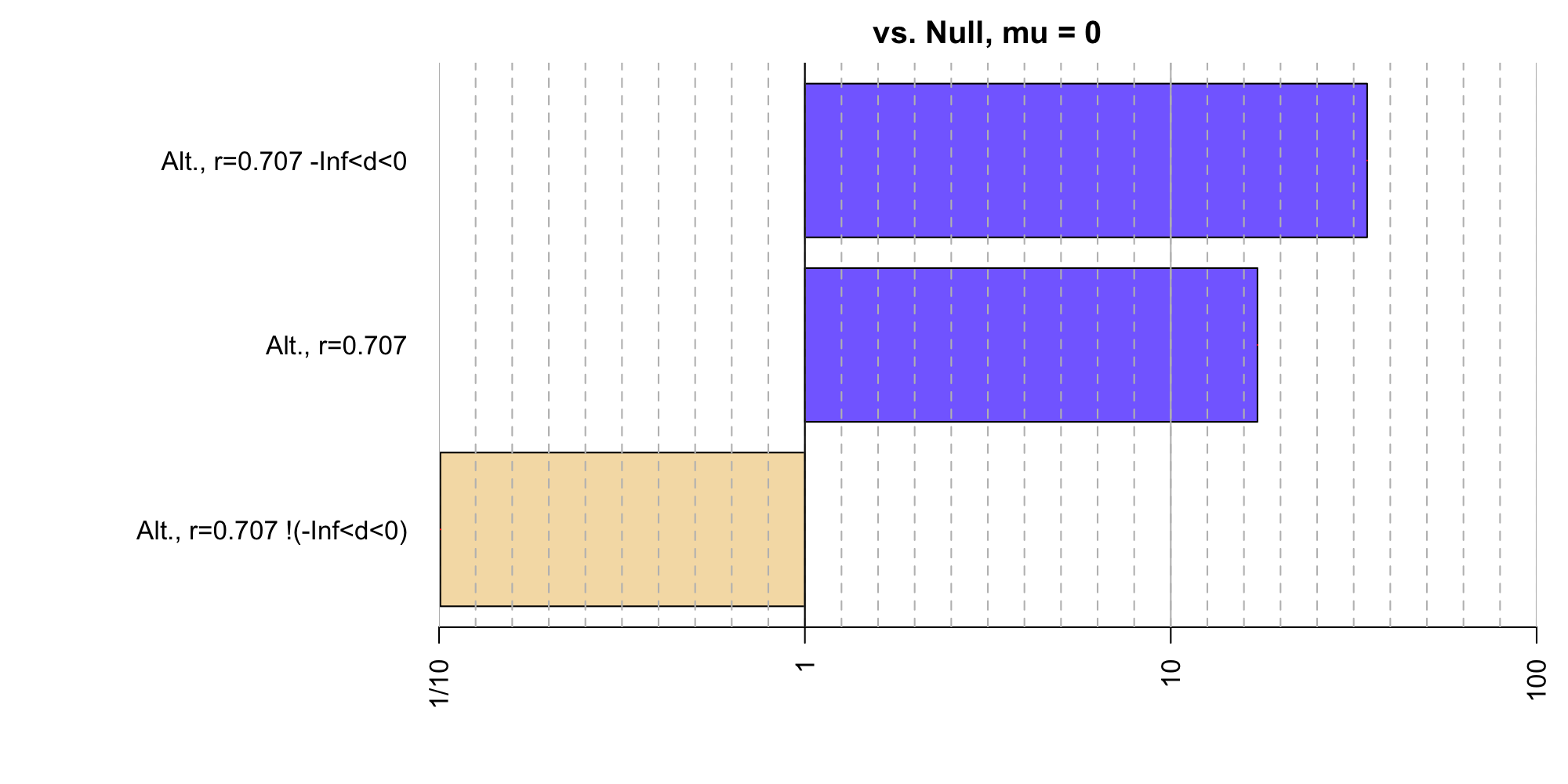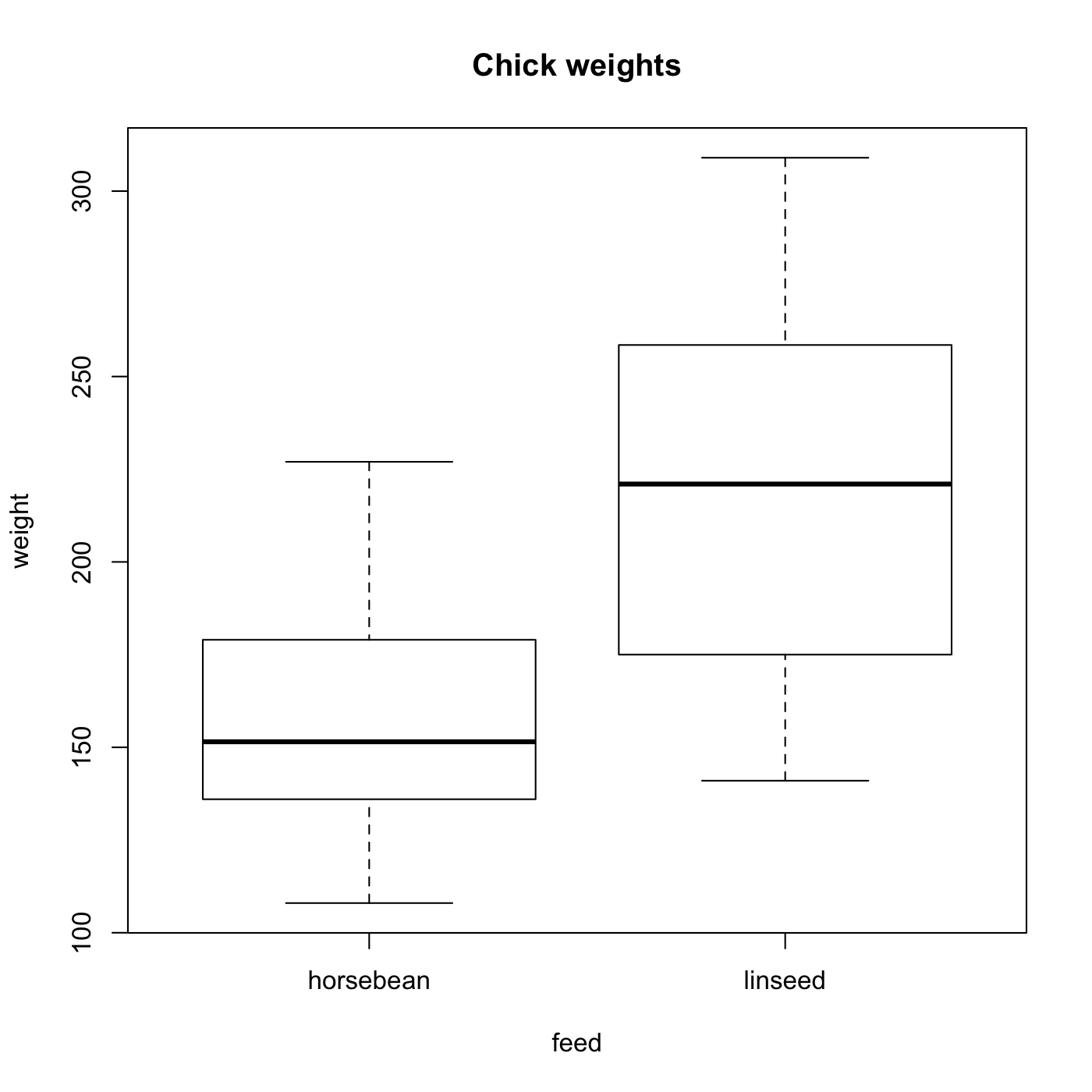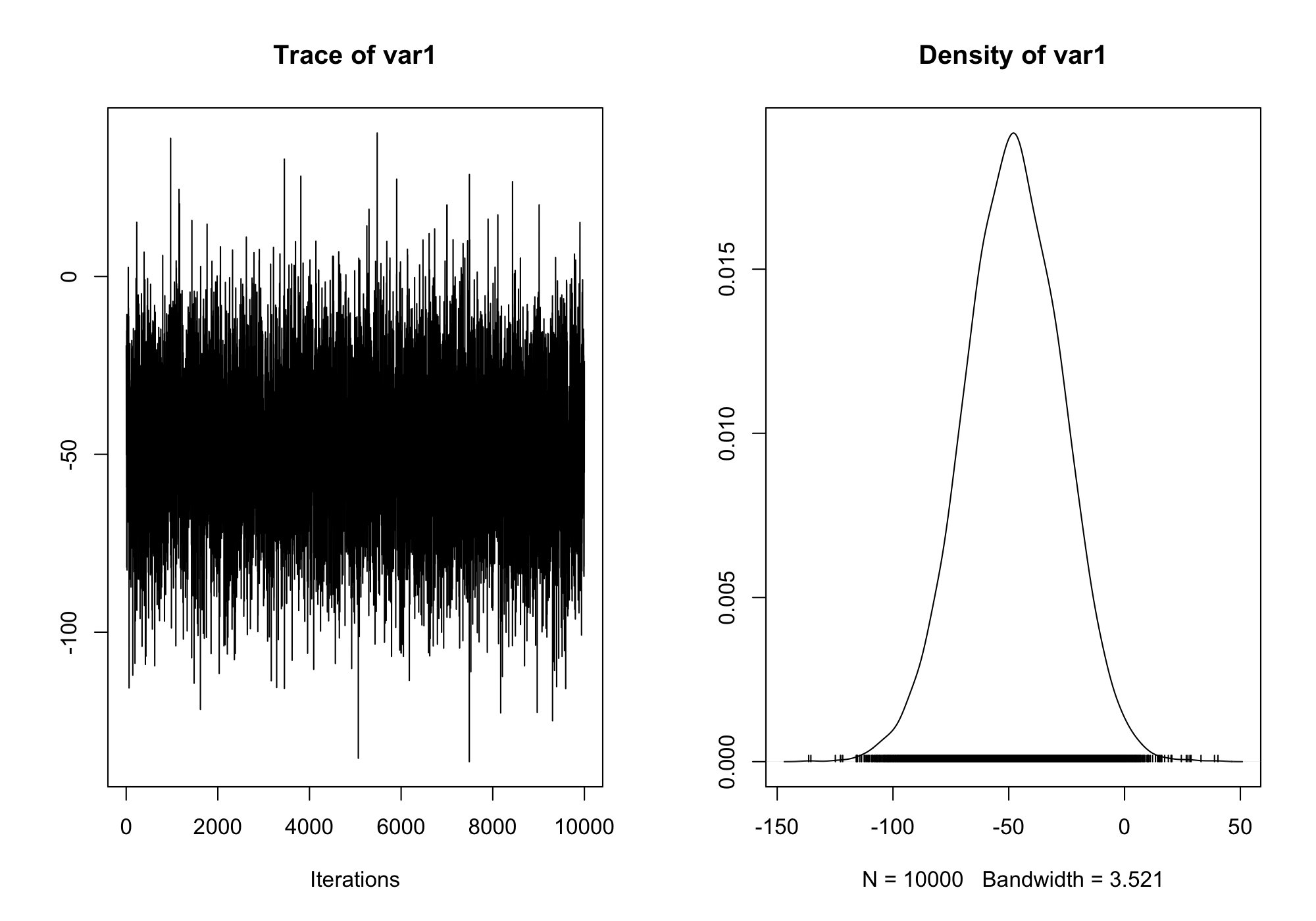# Using the 'BayesFactor' package, version 0.9.2+

## Richard D. Morey

Stable version: CRAN page - Package NEWS (including version changes)

Development version: Development page - Development package NEWS

### Introduction

The BayesFactor package enables the computation of Bayes factors in standard designs, such as one- and two- sample designs, ANOVA designs, and regression. The Bayes factors are based on work spread across several papers. This document is designed to show users how to compute Bayes factors using the package by example. It is not designed to present the models used in the comparisons in detail; for that, see the BayesFactor help and especially the references listed in this manual. Complete references are given at the end of this document.

If you need help or think you've found a bug, please use the links at the top of this document to contact the developers. When asking a question or reporting a bug, please send example code and data, the exact errors you're seeing (a cut-and-paste from the R console will work) and instructions for reproducing it. Also, report the output of BFInfo() and sessionInfo(), and let us know what operating system you're running.

The BayesFactor package must be installed and loaded before it can be used. Installing the package can be done in several ways and will not be covered here. Once it is installed, use the library function to load it:

library(BayesFactor)


This command will make the BayesFactor package ready to use.

### Some useful functions

The table below lists some of the functions in the BayesFactor package that will be demonstrated in this manual. For more complete help on the use of these functions, see the corresponding help() page in R.

Function Description
ttestBF Bayes factors for one- and two- sample designs
anovaBF Bayes factors comparing many ANOVA models
regressionBF Bayes factors comparing many linear regression models
generalTestBF Bayes factors for all restrictions on a full model (0.9.4+)
lmBF Bayes factors for specific linear models (ANOVA or regression)
correlationBF Bayes factors for linear correlations
proportionBF Bayes factors for tests of single proportions
contingencyTableBF Bayes factors for contingency tables
posterior Sample from the posterior distribution of the numerator of a Bayes factor object
recompute Recompute a Bayes factor or MCMC chain, possibly increasing the precision of the estimate
compare Compare two models; typically used to compare two models in BayesFactor MCMC objects

#### Functions to manipulate Bayes factor objects

The t test section below has examples showing how to manipulate Bayes factor objects, but all these functions will work with Bayes factors generated from any function in the BayesFactor package.

Function Description
/ Divide two Bayes factor objects to create new model comparisons, or invert with 1/
t “Flip” (transpose) a Bayes factor object
c Concatenate two Bayes factor objects together, assuming they have the same denominator
[ Use indexing to select a subset of the Bayes factors
plot plot a Bayes factor object
sort Sort a Bayes factor object
is.na Determine whether a Bayes factor object contains missing values
head,tail Return the n highest or lowest Bayes factor in an object
max, min Return the highest or lowest Bayes factor in an object
which.max,which.min Return the index of the highest or lowest Bayes factor
as.vector Convert to a simple vector (denominator will be lost!)
as.data.frame Convert to data.frame (denominator will be lost!)

### One- and two-sample designs (t tests)

The ttestBF function is used to obtain Bayes factors corresponding to tests of a single sample's mean, or tests that two independent samples have the same mean.

#### One-sample tests (and paired)

We use the sleep data set in R to demonstrate a one-sample t test. This is a paired design; for details about the data set, see ?sleep. One way of analyzing these data is to compute difference scores by subtracting a participant's score in one condition from their score in the other:

data(sleep)

## Compute difference scores
diffScores = sleep$extra[1:10] - sleep$extra[11:20]

t.test(diffScores)

##
##  One Sample t-test
##
## data:  diffScores
## t = -4, df = 9, p-value = 0.003
## alternative hypothesis: true mean is not equal to 0
## 95 percent confidence interval:
##  -2.46 -0.70
## sample estimates:
## mean of x
##     -1.58


We can do a Bayesian version of this analysis using the ttestBF function, which performs the “JZS” t test described by Rouder, Speckman, Sun, Morey, and Iverson (2009). In this model, the true standardized difference $$\delta=(\mu-\mu_0)/\sigma_\epsilon$$ is assumed to be 0 under the null hypothesis, and $$\text{Cauchy}(\text{scale}=r)$$ under the alternative. The default $$r$$ scale in BayesFactor for t tests is $$\sqrt{2}/2$$. See ?ttestBF for more details.

bf = ttestBF(x = diffScores)
## Equivalently:
## bf = ttestBF(x = sleep$extra[1:10],y=sleep$extra[11:20], paired=TRUE)
bf

## Bayes factor analysis
## --------------
##  Alt., r=0.707 : 17.3 ±0%
##
## Against denominator:
##   Null, mu = 0
## ---
## Bayes factor type: BFoneSample, JZS


The bf object contains the Bayes factor, and shows the numerator and denominator models for the Bayes factor comparison. In our case, the Bayes factor for the comparison of the alternative versus the null is 17.259. After the Bayes factor is a proportional error estimate on the Bayes factor.

There are a number of operations we can perform on our Bayes factor, such as taking the reciprocal:

1 / bf

## Bayes factor analysis
## --------------
##  Null, mu=0 : 0.0579 ±0%
##
## Against denominator:
##   Alternative, r = 0.707106781186548, mu =/= 0
## ---
## Bayes factor type: BFoneSample, JZS


or sampling from the posterior of the numerator model:

chains = posterior(bf, iterations = 1000)
summary(chains)

##
## Iterations = 1:1000
## Thinning interval = 1
## Number of chains = 1
## Sample size per chain = 1000
##
## 1. Empirical mean and standard deviation for each variable,
##    plus standard error of the mean:
##
##        Mean     SD Naive SE Time-series SE
## mu    -1.42  0.436   0.0138         0.0154
## sig2   2.02  1.157   0.0366         0.0395
## delta -1.11  0.427   0.0135         0.0162
## g      6.26 58.623   1.8538         1.8538
##
## 2. Quantiles for each variable:
##
##         2.5%    25%   50%    75%  97.5%
## mu    -2.289 -1.705 -1.43 -1.141 -0.597
## sig2   0.744  1.270  1.69  2.446  5.223
## delta -1.973 -1.383 -1.08 -0.813 -0.347
## g      0.176  0.592  1.13  2.928 33.734


The posterior function returns a object of type BFmcmc, which inherits the methods of the mcmc class from the coda package. We can thus use summary, plot, and other useful methods on the result of posterior. If we were unhappy with the number of iterations we sampled for chains, we can recompute with more iterations, and then plot the results:

chains2 = recompute(chains, iterations = 10000)
plot(chains2[,1:2])Directional hypotheses can also be tested with ttestBF (Morey & Rouder, 2011). The argument nullInterval can be passed as a vector of length 2, and defines an interval to compare to the point null. If null interval is defined, two Bayes factors are returned: the Bayes factor of the null interval against the alternative, and the Bayes factor of the complement of the interval to the point null.

Suppose, for instance, we wanted to test the one-sided hypotheses that $$\delta<0$$ versus the point null. We set nullInterval to c(-Inf,0):

bfInterval = ttestBF(x = diffScores, nullInterval=c(-Inf,0))
bfInterval

## Bayes factor analysis
## --------------
##  Alt., r=0.707 -Inf<d<0    : 34.4  ±0%
##  Alt., r=0.707 !(-Inf<d<0) : 0.101 ±0.06%
##
## Against denominator:
##   Null, mu = 0
## ---
## Bayes factor type: BFoneSample, JZS


We may not be interested in tests against the point null. If we are interested in the Bayes factor test that $$\delta<0$$ versus $$\delta>0$$ we can compute it using the result above. Since the object contains two Bayes factors, both with the same denominator, and $\left.\frac{A}{C}\middle/\frac{B}{C}\right. = \frac{A}{B},$ we can divide the two Bayes factors in bfInferval to obtain the desired test:

bfInterval / bfInterval

## Bayes factor analysis
## --------------
##  Alt., r=0.707 -Inf<d<0 : 341 ±0.06%
##
## Against denominator:
##   Alternative, r = 0.707106781186548, mu =/= 0 !(-Inf<d<0)
## ---
## Bayes factor type: BFoneSample, JZS


The Bayes factor is about 340.

When we have multiple Bayes factors that all have the same denominator, we can concatenate them into one object using the c function. Since bf and bfInterval both share the point null denominator, we can do this:

allbf = c(bf, bfInterval)
allbf

## Bayes factor analysis
## --------------
##  Alt., r=0.707             : 17.3  ±0%
##  Alt., r=0.707 -Inf<d<0    : 34.4  ±0%
##  Alt., r=0.707 !(-Inf<d<0) : 0.101 ±0.06%
##
## Against denominator:
##   Null, mu = 0
## ---
## Bayes factor type: BFoneSample, JZS


The object allbf now contains three Bayes factors, all of which share the same denominator. If you try to concatenate Bayes factors that do not share the same denominator, BayesFactor will return an error.

When you have a Bayes factor object with several numerators, there are several interesting ways to manipulate them. For instance, we can plot the Bayes factor object to obtain a graphical representation of the Bayes factors:

plot(allbf)We can also divide a Bayes factor object by itself — or by a subset of itself — to obtain pairwise comparisons:

bfmat = allbf / allbf
bfmat

##                            denominator
## numerator                   Alt., r=0.707 Alt., r=0.707 -Inf<d<0
##   Alt., r=0.707                   1.00000                0.50146
##   Alt., r=0.707 -Inf<d<0          1.99416                1.00000
##   Alt., r=0.707 !(-Inf<d<0)       0.00584                0.00293
##                            denominator
## numerator                   Alt., r=0.707 !(-Inf<d<0)
##   Alt., r=0.707                                   171
##   Alt., r=0.707 -Inf<d<0                          341
##   Alt., r=0.707 !(-Inf<d<0)                         1


The resulting object is of type BFBayesFactorList, and is a list of Bayes factor comparisons all of the same numerators compared to different denominators. The resulting matrix can be subsetted to return individual Bayes factor objects, or new BFBayesFactorLists:

bfmat[,2]

## Bayes factor analysis
## --------------
##  Alt., r=0.707             : 0.501   ±0%
##  Alt., r=0.707 -Inf<d<0    : 1       ±0%
##  Alt., r=0.707 !(-Inf<d<0) : 0.00293 ±0.06%
##
## Against denominator:
##   Alternative, r = 0.707106781186548, mu =/= 0 -Inf<d<0
## ---
## Bayes factor type: BFoneSample, JZS

bfmat[1,]

##                denominator
## numerator       Alt., r=0.707 Alt., r=0.707 -Inf<d<0
##   Alt., r=0.707             1                  0.501
##                denominator
## numerator       Alt., r=0.707 !(-Inf<d<0)
##   Alt., r=0.707                       171


and they can also be transposed:

bfmat[,1:2]

##                            denominator
## numerator                   Alt., r=0.707 Alt., r=0.707 -Inf<d<0
##   Alt., r=0.707                   1.00000                0.50146
##   Alt., r=0.707 -Inf<d<0          1.99416                1.00000
##   Alt., r=0.707 !(-Inf<d<0)       0.00584                0.00293

t(bfmat[,1:2])

##                         denominator
## numerator                Alt., r=0.707 Alt., r=0.707 -Inf<d<0
##   Alt., r=0.707                   1.00                  0.501
##   Alt., r=0.707 -Inf<d<0          1.99                  1.000
##                         denominator
## numerator                Alt., r=0.707 !(-Inf<d<0)
##   Alt., r=0.707                                171
##   Alt., r=0.707 -Inf<d<0                       341


If these values are desired in matrix form, the as.matrix function can be used to obtain a matrix.

#### Two-sample test (independent groups)

The ttestBF function can also be used to compute Bayes factors in the two sample case as well. We use the chickwts data set to demonstrate the two-sample t test. The chickwts data set has six groups, but we reduce it to two for the demonstration.

data(chickwts)

## Restrict to two groups
chickwts = chickwts[chickwts$feed %in% c("horsebean","linseed"),] ## Drop unused factor levels chickwts$feed = factor(chickwts\$feed)

## Plot data
plot(weight ~  feed, data = chickwts, main = "Chick weights")Chick weight appears to be affected by the feed type.

## traditional t test
t.test(weight ~ feed, data = chickwts, var.eq=TRUE)

##
##  Two Sample t-test
##
## data:  weight by feed
## t = -3, df = 20, p-value = 0.008
## alternative hypothesis: true difference in means is not equal to 0
## 95 percent confidence interval:
##  -100.2  -16.9
## sample estimates:
## mean in group horsebean   mean in group linseed
##                     160                     219


We can also compute the corresponding Bayes factor. There are two ways of specifying a two-sample test: the formula interface and through the x and y arguments. We show the formula interface here:

## Compute Bayes factor
bf = ttestBF(formula = weight ~ feed, data = chickwts)
bf

## Bayes factor analysis
## --------------
##  Alt., r=0.707 : 5.98 ±0%
##
## Against denominator:
##   Null, mu1-mu2 = 0
## ---
## Bayes factor type: BFindepSample, JZS


As before, we can sample from the posterior distribution for the numerator model:

chains = posterior(bf, iterations = 10000)
plot(chains[,2])Note that the samples assume an (equivalent) ANOVA model; see ?ttestBF and for notes on the differences in interpretation of the $$r$$ scale parameter between the two models.

### Meta-analytic t tests (0.9.8+)

Rouder and Morey (2011; link) discuss a meta-analytic extension of the $$t$$ test, whereby multiple $$t$$ statistics, along with their corresponding sample sizes, are combined in a single meta-analytic analysis. The $$t$$ statistics are assumed to arise from a a common effect size $$\delta$$. The prior for the effect size $$\delta$$ is the same as that for the $$t$$ tests described above.

The meta.ttestBF function is used to perform meta-analytic $$t$$ tests. It requires as input a vector of $$t$$ statistics, and one or two vectors of sample sizes (arguments n1 and n2). For a set of one-sample $$t$$ statistics, n1 should be provided; for two-sample analyses, both n1 and n2 should be provided.

As an example, we will replicate the analysis of Rouder & Morey (2011), using $$t$$ statistics from Bem (2010; see Rouder & Morey for reference). We begin by defining the one-sample $$t$$ statistics and sample sizes:

## Bem's t statistics from four selected experiments
t = c(-.15, 2.39, 2.42, 2.43)
N = c(100, 150, 97, 99)


Rouder and Morey opted for a one-sided analysis, and used an $$r$$ scale parameter of 1 (instead of the current default in BayesFactor of $$\sqrt{2}/2$$).

bf = meta.ttestBF(t=t, n1=N, nullInterval=c(0,Inf), rscale=1)
bf

## Bayes factor analysis
## --------------
##  Alt., r=1 0<d<Inf    : 38.7    ±0%
##  Alt., r=1 !(0<d<Inf) : 0.00803 ±0.56%
##
## Against denominator:
##   Null, d = 0
## ---
## Bayes factor type: BFmetat, JZS


Notice that as above, the analysis yields a Bayes factor for our selected interval against the null, as well as the Bayes factor for the complement of the interval against the null.

We can also sample from the posterior distribution of the standardized effect size $$\delta$$, as above, using the posterior function:

## Do analysis again, without nullInterval restriction
bf = meta.ttestBF(t=t, n1=N, rscale=1)

## Obtain posterior samples
chains = posterior(bf, iterations = 10000)

## Independent-candidate M-H acceptance rate: 98%

plot(chains)